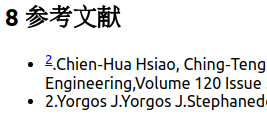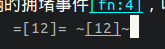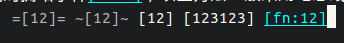# org-mode 如何输出?请问org-mode想写论文的参考文献，可无法输出正常的值，导出html被干掉了。。 试过了，不行。。。

1 个赞

footnote 的标记是由 `org-footnote-re` 这个变量控制的，就是一个大正则。可以修改这个变量

``````(setq org-footnote-re
(concat "\\[\\(?:"
;; Match inline footnotes.
(org-re "fn:\\([-_[:word:]]+\\)?:\\|")
;; Match other footnotes.
;; "\\(?:\\([0-9]+\\)\\]\\)\\|"
(org-re "\\(fn:[-_[:word:]]+\\)")
"\\)"))

(setq org-footnote-definition-re
(org-re "^\\[\\(fn:[-_[:word:]]+\\)\\]"))
``````

1 个赞

``````a ~~ a
a
~~
a
a == a
a
==
a
``````

``````a  a a  a a  a a  a
``````

``````<p>
a <code></code> a
a
<code></code>
a
a <code></code> a
a
<code></code>
a
</p>
``````非常不错，两个都可以哈哈！谢谢看来这才是究极方案呀，直接抄到我的配置里，谢谢大师～